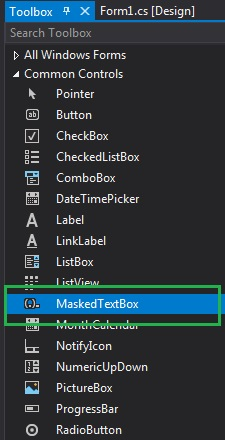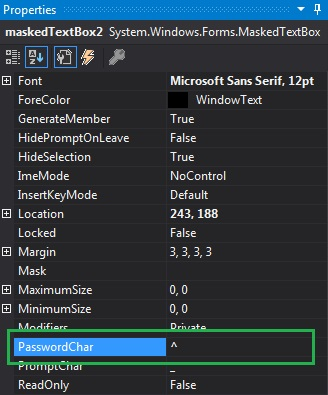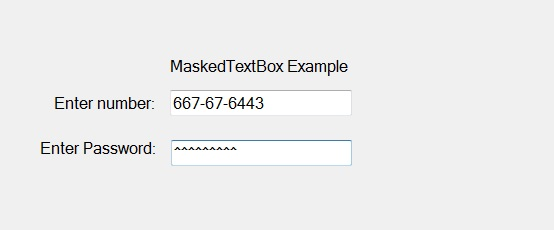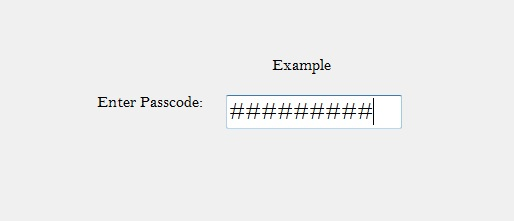• Last Updated : 26 Jul, 2019

In C#, MaskedTextBox control gives a validation procedure for the user input on the form like date, phone numbers, etc. Or in other words, it is used to provide a mask which differentiates between proper and improper user input. In MaskedTextBox control, you are allowed to set the character which displays when we enter sensitive data like password in the MaskedTextBox using PasswordChar Property provided by the MaskedTextBox control.
If the value of this property is set to non-null character, then the MaskedTextBox will display this character for all input, and if the value of this property is set to null, then it will not display anything in the MaskedTextBox control. You can set this property in two different ways:

1. Design-Time: It is the easiest way to set the value of PasswordChar property of MaskedTextBox control as shown in the following steps:

• Step 1: Create a windows form as shown in the below image:
Visual Studio -> File -> New -> Project -> WindowsFormApp• Step 2: Next, drag and drop the MaskedTextBox control from the toolbox on the form as shown in the below image:• Step 3: After drag and drop you will go to the properties of the MaskedTextBox and set the value of PasswordChar property of MaskedTextBox control as shown in the below image:Output:2. Run-Time: It is a little bit trickier than the above method. In this method, you can set the value of PasswordChar property of the MaskedTextBox control programmatically with the help of given syntax:

`public char PasswordChar { get; set; }`

Here, the Char represents the password character value. The following steps show how to set the value of PasswordChar property of the MaskedTextBox control dynamically:

```// Creating a MaskedTextBox
```
```// Setting the PasswordChar property
```
• Step 3: And last add this MaskedTextBox control to the form using the following statement:
```// Adding MaskedTextBox control on the form
```

Example:

 `using` `System;``using` `System.Collections.Generic;``using` `System.ComponentModel;``using` `System.Data;``using` `System.Drawing;``using` `System.Linq;``using` `System.Text;``using` `System.Threading.Tasks;``using` `System.Windows.Forms;`` ` `namespace` `WindowsFormsApp39 {`` ` `public` `partial` `class` `Form1 : Form {`` ` `    ``public` `Form1()``    ``{``        ``InitializeComponent();``    ``}`` ` `    ``private` `void` `Form1_Load(``object` `sender, EventArgs e)``    ``{``        ``// Creating and setting the ``        ``// properties of the Label``        ``Label l1 = ``new` `Label();``        ``l1.Location = ``new` `Point(413, 98);``        ``l1.Size = ``new` `Size(176, 20);``        ``l1.Text = ``" Example"``;``        ``l1.Font = ``new` `Font(``"Bell MT"``, 12);`` ` `        ``// Adding label on the form``        ``this``.Controls.Add(l1);`` ` `        ``// Creating and setting the ``        ``// properties of the Label``        ``Label l2 = ``new` `Label();``        ``l2.Location = ``new` `Point(242, 135);``        ``l2.Size = ``new` `Size(126, 20);``        ``l2.Text = ``"Enter Passcode:"``;``        ``l2.Font = ``new` `Font(``"Bell MT"``, 12);`` ` `        ``// Adding label on the form``        ``this``.Controls.Add(l2);`` ` `        ``// Creating and setting the ``        ``// properties of MaskedTextBox``        ``MaskedTextBox m = ``new` `MaskedTextBox();``        ``m.Location = ``new` `Point(374, 137);``        ``m.Mask = ``"000000000"``;``        ``m.Size = ``new` `Size(176, 20);``        ``m.Name = ``"MyBox"``;``        ``m.BorderStyle = BorderStyle.Fixed3D;``        ``m.PasswordChar = ``'#'``;``        ``m.Font = ``new` `Font(``"Bell MT"``, 18);`` ` `        ``// Adding MaskedTextBox ``        ``// control on the form``        ``this``.Controls.Add(m);``    ``}``}``}`

Output:My Personal Notes arrow_drop_up